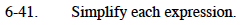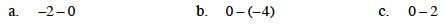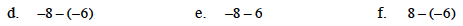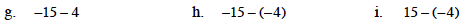### Home > MC1 > Chapter 6 > Lesson 6.1.4 > Problem6-41

6-41.Subtracting zero from another integer will not have an impact. However, subtracting an integer from zero will make a difference in simplifying the expression. Keep this in mind for parts (a), (b), and (c).Remember to pay close attention to each of the signs. Subtracting negatives can be tricky!

−2

14Be sure to show your work! And remember, subtracting integers is like taking steps backwards, but subtracting negative integers is like moving forward.

−19

−11

19